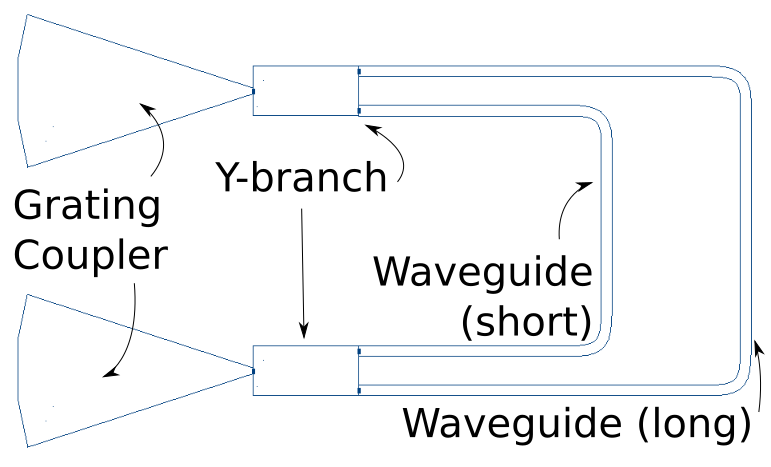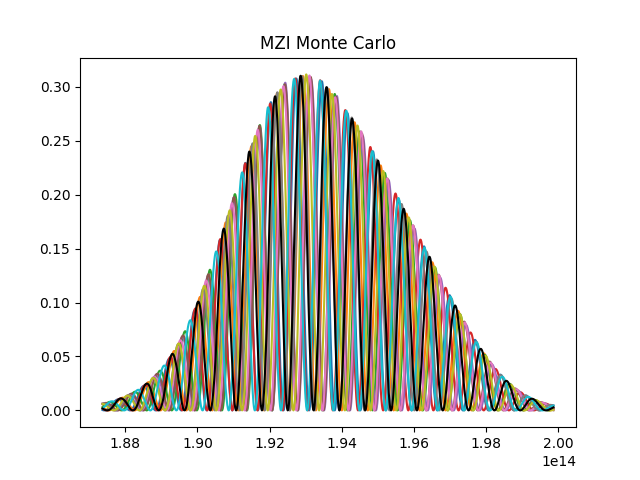# Mach-Zehnder Interferometer¶

In this tutorial, we’ll define and simulate a simple circuit known as a Mach-Zender Interferometer (MZI). This tutorial will walk through the code found in `examples/mzi.py` of the Simphony repository. We expect you to have read the previous tutorial, Introduction to Simphony.

## Deconstructing the MZI¶

In an MZI, light entering the circuit is split and travels down two paths of differing lengths. When the light is recombined, it interferes, and the output is frequency-dependent.

The MZI we’ll create can be broken down into constituent parts. Simphony includes models for these constituent parts below:The grating couplers are the input and output for light in the circuit. The Y-branch can split and recombine light, and because the waveguides which carry light across the circuit are varying length, this produces interference when the light is recombined at the second Y-branch. We can now begin defining our circuit in Simphony using the components we have identified.

## Defining The Circuit¶

First we need to import the necessary Simphony modules. The `siepic` model library, the `SweepSimulator` and the `MonteCarloSweepSimulator` will be all that we need. We will also import `matplotlib.pyplot`, from outside of Simphony, to view the results of our simulation.

```import matplotlib.pyplot as plt

from simphony.libraries import siepic
from simphony.simulators import MonteCarloSweepSimulator, SweepSimulator
```

We then create all the components identified earlier. These include the grating couplers, the Y-branches, and the waveguides (which can be defined at any arbitrary length, on the condition that the two lengths are different).

```gc_input = siepic.GratingCoupler()
y_splitter = siepic.YBranch()
wg_long = siepic.Waveguide(length=150e-6)
wg_short = siepic.Waveguide(length=50e-6)
y_recombiner = siepic.YBranch()
gc_output = siepic.GratingCoupler()
```

Once we have our components, we can connect them. We can reference the diagram above on which connections to make.

```# next we connect the components to each other
# you can connect pins directly:
y_splitter["pin1"].connect(gc_input["pin1"])

# or connect components with components:
# (when using components to make connections, their first unconnected pin will
# be used to make the connection.)
y_splitter.connect(wg_long)

# or any combination of the two:
y_splitter["pin3"].connect(wg_short)
# y_splitter.connect(wg_short["pin1"])

# when making multiple connections, it is often simpler to use `multiconnect`
# multiconnect accepts components, pins, and None
# if None is passed in, the corresponding pin is skipped
y_recombiner.multiconnect(gc_output, wg_short, wg_long)
```

Note

There are several different ways of connecting components in Simphony, and many are demonstrated in this example, with comments included.

You may also wish to rename pins for better semantics when writing your own circuit. This can be done with `component.rename_pins('new_pin1', 'new_pin2', ...)`, on any of the components we have created.

`rename_pins` can be called on a model itself. This will create default names for any new component you instantiate of that model.

These are all the connections required to define our circuit. The next step will be to run the simulations.

## Sweep Simulation¶

First, we’ll do a standard sweep simulation. We define the simulator, the frequency range to run, and then connect it to the input and output of our circuit:

```simulator = SweepSimulator(1500e-9, 1600e-9)
simulator.multiconnect(gc_input, gc_output)
```

Now we simulate. We hand the results over to `matplotlib` for a graph visualization (see matplotlib docs for how it works).

```f, p = simulator.simulate()
plt.plot(f, p)
plt.title("MZI")
plt.tight_layout()
plt.show()
```

When you run your MZI python file, it should bring up a graph showing something similar to this:## Monte-Carlo Simulation¶

Let’s also run a Monte-Carlo simulation. This type of simulation estimates manufacturing variability and margin of error found on real silicon circuits. Disconnect the previous simulator to connect the new simulator:

```simulator.disconnect()
simulator = MonteCarloSweepSimulator(1500e-9, 1600e-9)
simulator.multiconnect(gc_input, gc_output)
```

Then we run the Monte-Carlo simulation several times, plotting each curve. We will now see several, slightly different curves on our graph due to random variation.

```results = simulator.simulate(runs=10)
for f, p in results:
plt.plot(f, p)
```

Finally, we can plot the first simulation again with black, to make sure it displays on top. We want this because the first simulation records results for ideal conditions.

```f, p = results
plt.plot(f, p, "k")
plt.title("MZI Monte Carlo")
plt.tight_layout()
plt.show()
```

You should see something similar to this graph when you run your MZI now:We have now defined and simulated our MZI! This completes our tutorial.